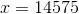## Example Questions

### Example Question #1 : How To Find The Amount Of Sales Tax

Beth purchased $21.25 worth of groceries. The sales tax rate is 7.5%. What was her total bill? Possible Answers:$23.41

$23.79$22.84

$21.93$22.11

$22.84 Explanation: The tax is calculated by 0.075 * 21.25 = 1.59375 or$1.59. So the total bill is the cost of items purchased plus tax, which is $21.25 +$1.59 = $22.84. ### Example Question #2 : How To Find The Amount Of Sales Tax The last week of August is tax-free week. If the standard tax rate is 8.25%, how much money would a shopper save buying a$50 dress and a $30 pair of shoes during tax free week? Possible Answers:$2.47

$7.25$4.13

$8.00$6.60

$6.60 Explanation: Tax = Total Cost * 0.0825 = (50 + 30) * 0.0825 = (80) x 0.0825 = 6.6 =$6.60

### Example Question #3 : How To Find The Amount Of Sales Tax

If a stick of deodorant costs $4.29 with a sales tax of 8.75%. What will the total cost of the item be? (round to the nearest cent) Possible Answers:$6.47

$4.67$4.76

$4.66$4.37

$4.67 Explanation: To solve for the tax charged multiply 0.0875 by the sales price of$4.29.

This is $0.375375. Add this to the original amount of$4.29.

The answer is 4.665375. Rounded to the nearest cent is $4.67 ### Example Question #4 : How To Find The Amount Of Sales Tax On a grocery shopping trip you spent$15 on meat, $5 on cheese, and$25 on produce.  If the total bill was $48.15, what is the rate of sales tax on the food? Possible Answers: 0.75% 7.5% 0.7% 6.5% 7% Correct answer: 7% Explanation: Tax = Total Bill - Cost of Food = 48.15 – (15 + 5 + 25) =$3.15

Percentage of Tax = Tax / Cost of Food = 3.15/ (15 + 5 + 25) = 3.15 / 45 = 0.07 = 7%

### Example Question #5 : How To Find The Amount Of Sales Tax

Regular sales tax is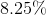.  Saturday is a tax free day that applies to clothes.  You want to buy a pair of jeans that are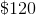, a pair of shoes that are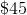, and a new shirt that costs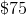.  How much money will you save if you want to go shopping on Saturday?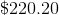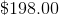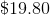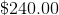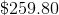Explanation:

Total cost = $120 +$45 + $75 =$240

Cost of Sales Tax = 8.25% ($240) = 0.0825 ($240) = $19.80 ### Example Question #6 : How To Find The Amount Of Sales Tax Jim buys a pair of shoes for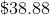, including tax. If the shoes were priced at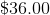, what percentage tax rate was he charged? Possible Answers: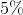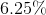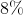%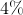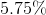Correct answer:% Explanation: Step 1: Find the amount of tax tax paid Jim paidincluding tax for shoes that were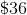pre-tax. Therefore: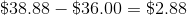Jim paid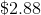in tax. Step 2: Figure out the tax rate (What percentage ofis?) Set up a proportion and solve for: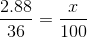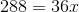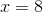Therefore, Jim paid antax on the shoes he purchased. ### Example Question #7 : How To Find The Amount Of Sales Tax If a given locality charges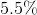sales tax, what is the total price for purchasing an item that costs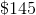before tax? Round to the nearest cent. Possible Answers: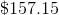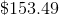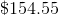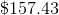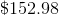Correct answer:Explanation: If a given locality chargessales tax, what is the total price for purchasing an item that costsbefore tax? First, convertto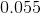. Then, multiply this by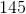to get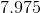dollars. Add this to the original price to get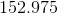. This rounds to. Another way to do this is to multiply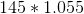to get the same amount. ### Example Question #8 : How To Find The Amount Of Sales Tax Sarah is buying a shirt that she likes. The shirt costs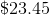but there is an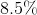sales tax. How much does Sarah pay for the shirt in total, after sales tax is added? (Round to the nearest cent.) Possible Answers: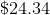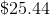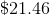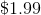Correct answer:Explanation: To find how much total is payed for an item after sales tax, conver the sales tax to a decimal and add it to 1, then multiply that total to the price of the item.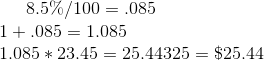### Example Question #9 : How To Find The Amount Of Sales Tax Before tax is calculated, Billy's bill at a restaurant is$50.00. If sales tax is 8%, how much tax does he pay?

$8.00$58.00

$4.00$50.00

$54.00 Correct answer:$4.00

Explanation:

You evaluate $50.00 x (0.08) =$4.00

### Example Question #10 : How To Find The Amount Of Sales Tax

The total cost of a new boat, inclusive of sales tax, was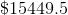. If the sales tax rate is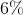, what was the original price of this boat before sales tax?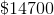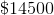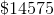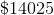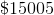Explanation:

The easiest way to do this is to write out the equation as though you were solving from the original price to find the final price. You would know that: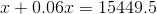or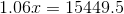Solving for, you get: